Manufacturing problems

Chapter 12 Class 12 Linear Programming
Concept wise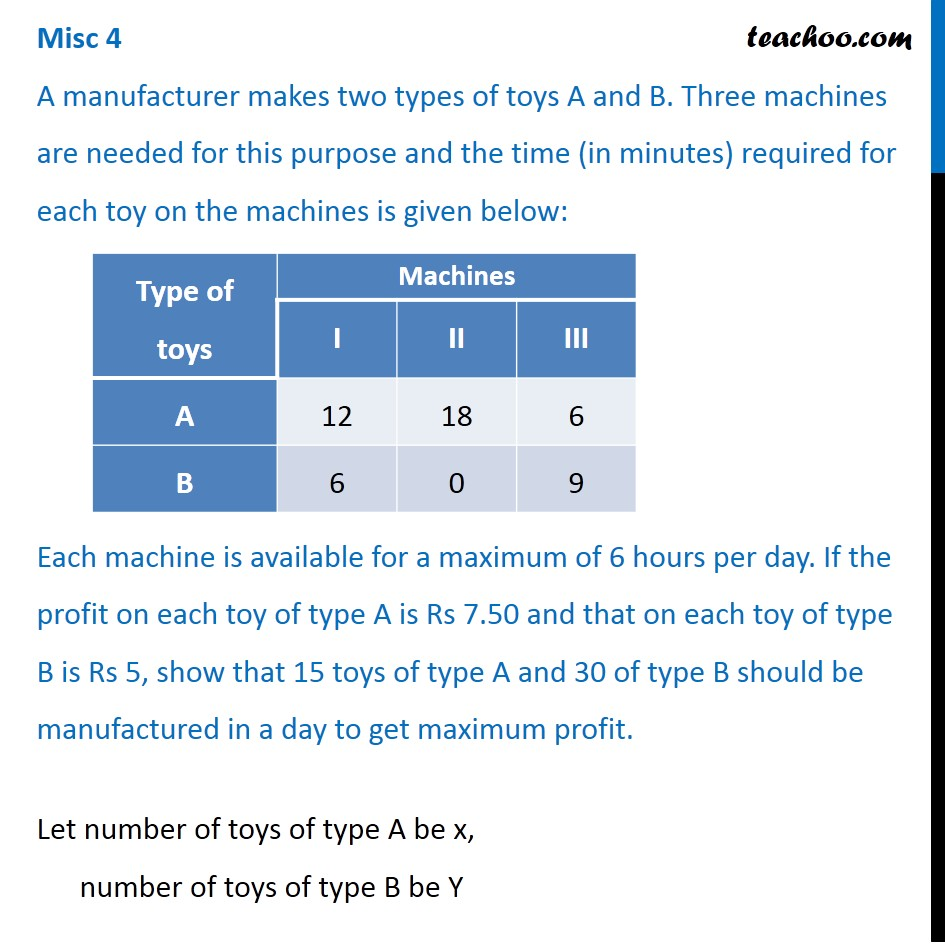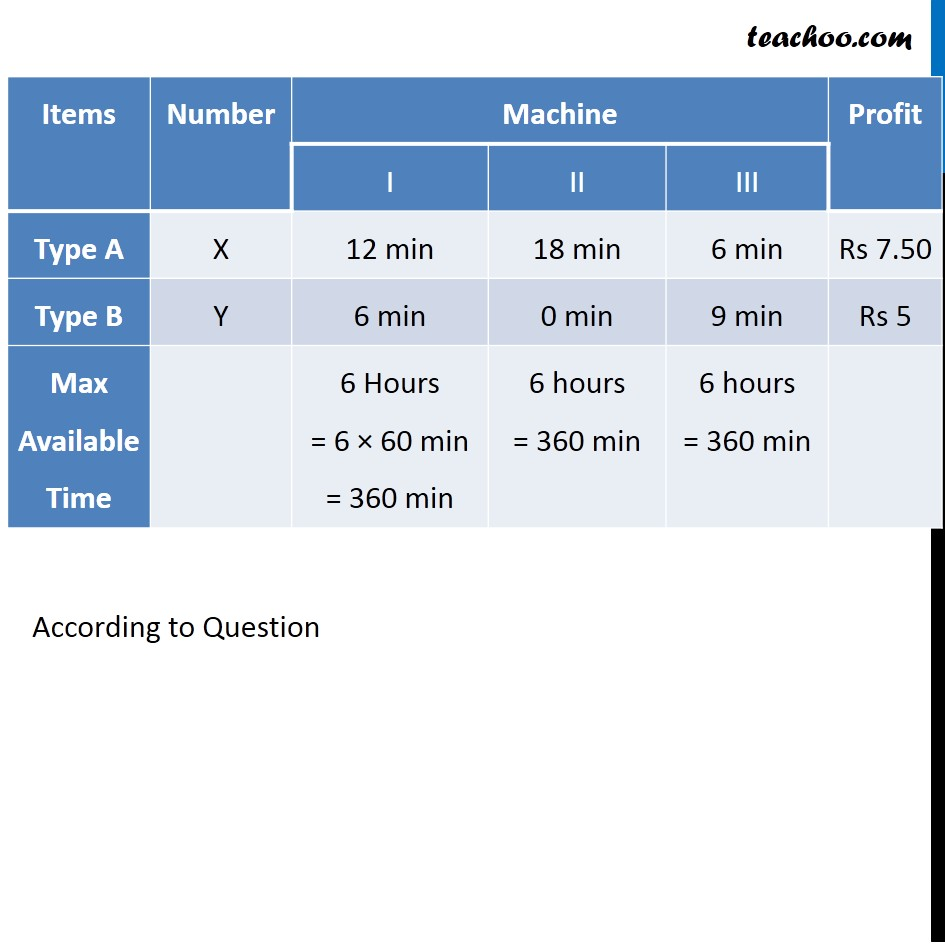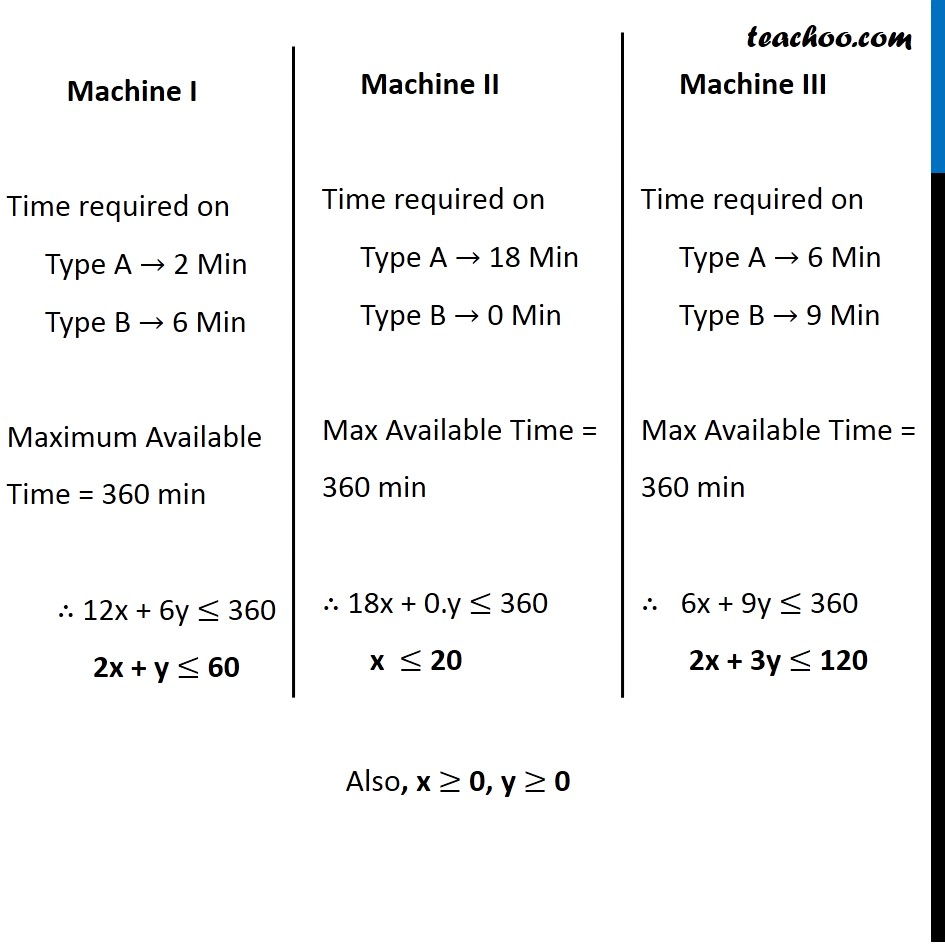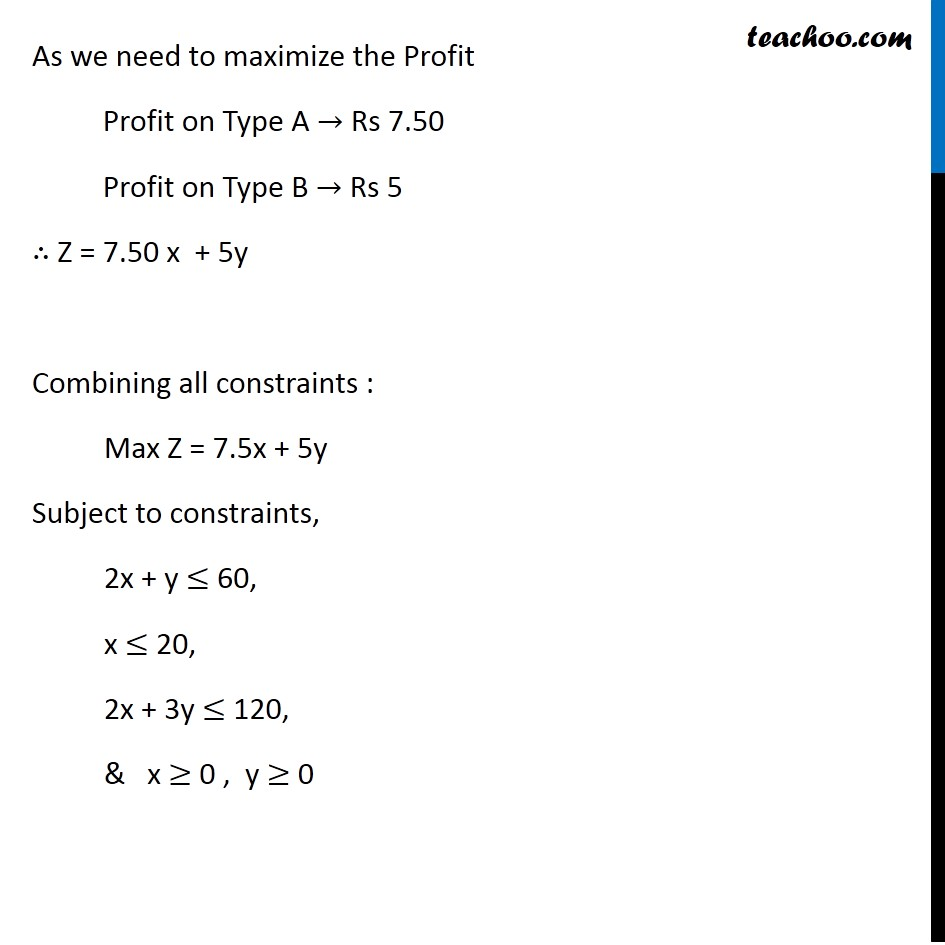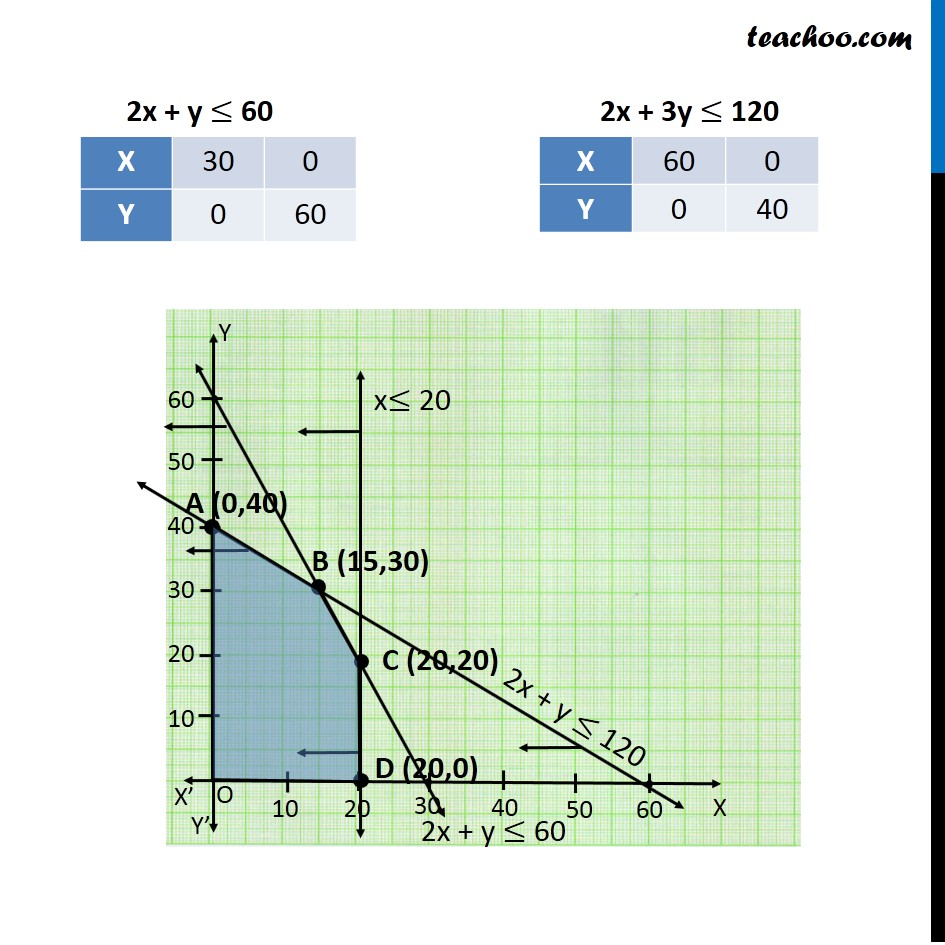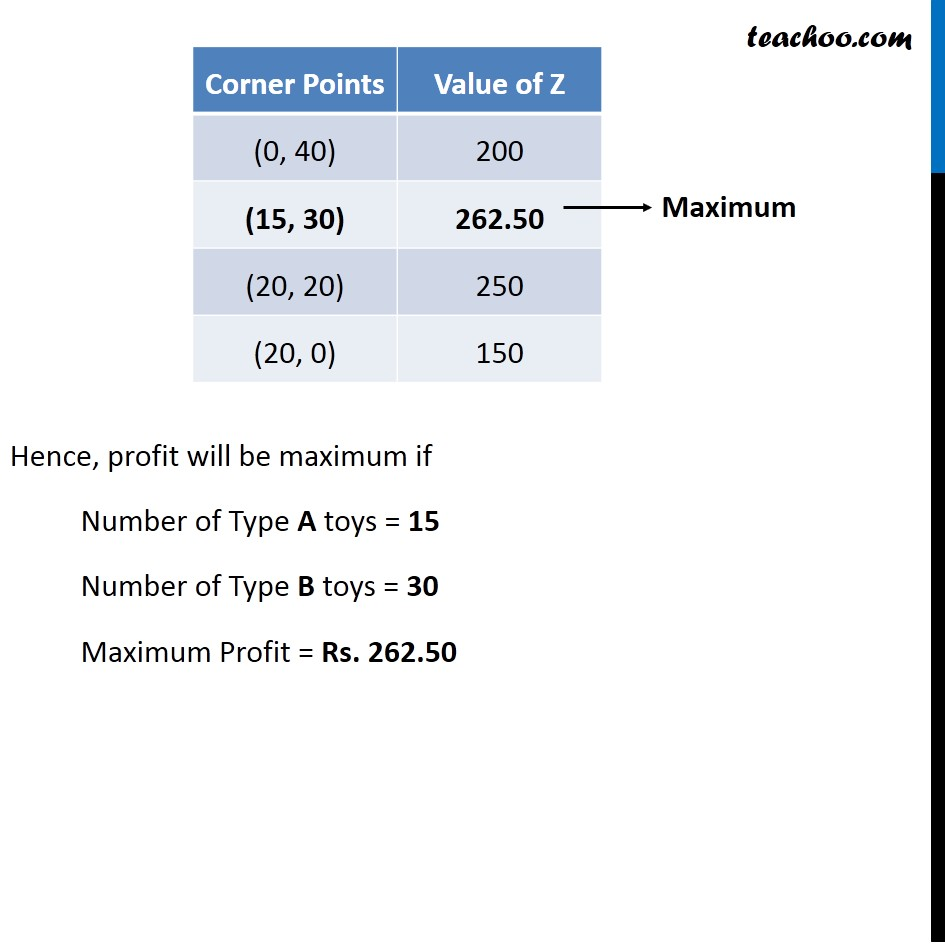Introducing your new favourite teacher - Teachoo Black, at only ₹83 per month

### Transcript

Misc 4 A manufacturer makes two types of toys A and B. Three machines are needed for this purpose and the time (in minutes) required for each toy on the machines is given below: Each machine is available for a maximum of 6 hours per day. If the profit on each toy of type A is Rs 7.50 and that on each toy of type B is Rs 5, show that 15 toys of type A and 30 of type B should be manufactured in a day to get maximum profit.Let number of toys of type A be x, number of toys of type B be Y According to Question According to Question Machine II Time required on Type A → 18 Min Type B → 0 Min Max Available Time = 360 min ∴ 18x + 0.y ≤ 360 x ≤ 20 Machine III Time required on Type A → 6 Min Type B → 9 Min Max Available Time = 360 min ∴ 6x + 9y ≤ 360 2x + 3y ≤ 120 As we need to maximize the Profit Profit on Type A → Rs 7.50 Profit on Type B → Rs 5 ∴ Z = 7.50 x + 5y Combining all constraints : Max Z = 7.5x + 5y Subject to constraints, 2x + y ≤ 60, x ≤ 20, 2x + 3y ≤ 120, & x ≥ 0 , y ≥ 0 Hence, profit will be maximum if Number of Type A toys = 15 Number of Type B toys = 30 Maximum Profit = Rs. 262.50 Hence, profit will be maximum if Number of Type A toys = 15 Number of Type B toys = 30 Maximum Profit = Rs. 262.50